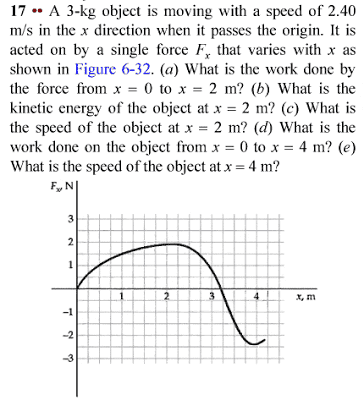# Work with variable force HELP ME!

## Homework StatementThe solutions are:
a)2,75J
b)11,4J
c)2,76m/s
d)3,5J
e)2,84m/s

## The Attempt at a Solution

I'm having problemas trying to integrate to find the letter A (the work)! I just can't put the values that match with x=0 (this one I know hehehe) and x=2(this one I don't know =/ )

## Answers and Replies

You are correct that work done is an integral of Force with respect to distance.
As you are given a graph of F against x with squares on it, what would be the simplest way to find (estimate) the value of this integral? Say, between x=0 and x=2?